Courses

# NCERT Chapter Notes 6 - The Triangle and its Properties, Mathematics, Class 7 | EduRev Notes

## Mathematics (Maths) Class 7

Created by: Praveen Kumar

## Class 7 : NCERT Chapter Notes 6 - The Triangle and its Properties, Mathematics, Class 7 | EduRev Notes

The document NCERT Chapter Notes 6 - The Triangle and its Properties, Mathematics, Class 7 | EduRev Notes is a part of the Class 7 Course Mathematics (Maths) Class 7.
All you need of Class 7 at this link: Class 7

6. The Triangle and its Properties

Triangles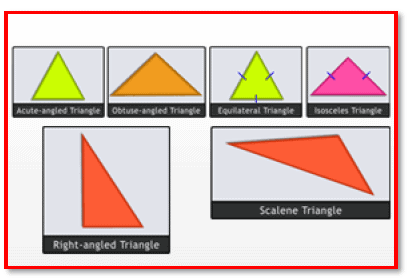A triangle is said to be equilateral if each one of its sides is of the same length and each of one its angles measures

The Triangle and Its Properties

A triangle is a closed figure made of three line segments. Every triangle has three sides, three angles, and three vertices. These are known as the parts of a triangle. The sides and the angles of every triangle differ from one another; therefore, they do not look alike.

Triangles can be classified based on their sides and angles.

♦ Based on their sides, there are equilateral, isosceles and scalene triangles.

♦ Based on their angles, there are acute, obtuse and right-angled triangles.

Equilateral triangle: A triangle in which all the sides are equal is called an equilateral triangle. All the three angles of an equilateral triangle are also equal, and each measures 60°.

Isosceles triangle: A triangle in which any two sides are equal is called an isosceles triangle. In an isosceles triangle, the angles opposite the equal sides are called the base angles, and they are equal.

Scalene triangle: A triangle in which no two sides are equal is called an Scalene triangle.

Acute-angled triangle: A triangle with all its angles less than 90° is known as an acute-angled triangle.

Page 20

Obtuse-angled triangle: A triangle with one of its angles more than 90° and less than 180° is known as an obtuse-angled triangle.

Right-angled triangle: A triangle with one of its angles equal to 90° is known as a right-angled triangle. The side opposite the 90° angle is called the hypotenuse, and is the longest side of the triangle.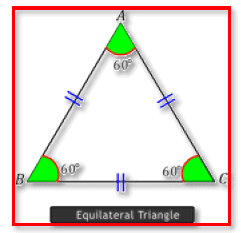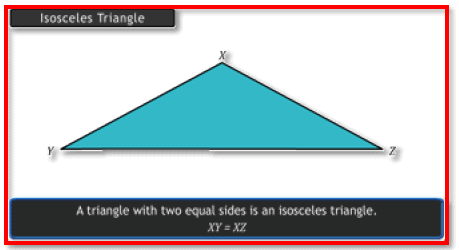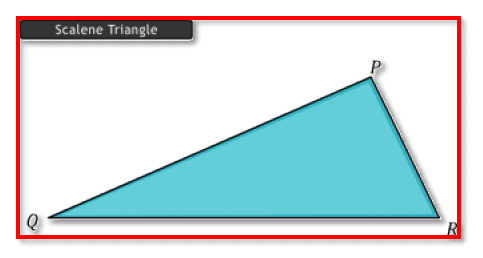Mark the mid-point of the side of a triangle, and join it to its opposite vertex. This line segment is called a median. It is defined as a line segment drawn from a vertex to the mid-point of the opposite side. You can draw three medians to a given triangle. The medianspass through a common point. Hence, the mediansof a triangle are concurrent. This point of concurrence is called the centroid, and is denoted by G. The centroidand medians of a triangle always lie inside the triangle. The centroid of a triangle divides the median in the ratio 2:1.

Page 21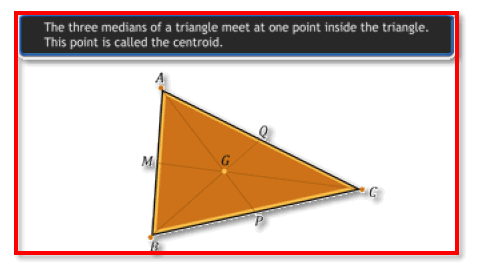Altitude: The altitude of a triangle is a line segment drawn from a vertex and is perpendicular to the opposite side. A triangle has three altitudes. The altitudes of a triangle are concurrent. The point of concurrence is called the orthocentre, and is denoted by O.

The altitude and orthocentre of a triangle need not lie inside the triangle.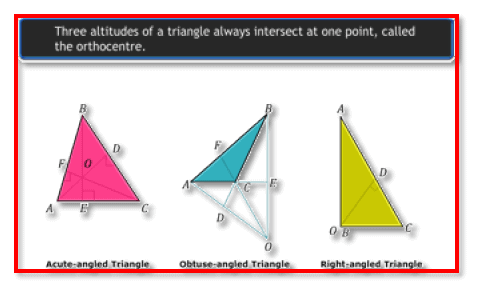Page 22

Properties of Triangles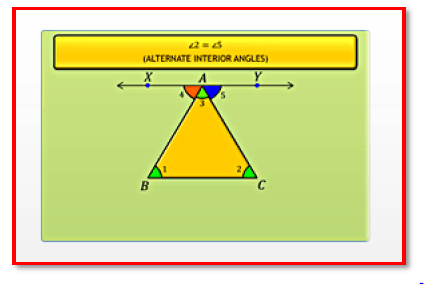The sum of the three angles in a triangle

Angle sum property:

The sum of the three angles in a triangle is equal to 180°

Eg: If ∠A,∠B and ∠C are the angles of a triangle, then

Suppose a line XY is parallel to side BC. AB is a transversal that cuts line XY and AB, at A and B, respectively. As the alternate interior angles are equal, .∠1 =∠4 Also, ∠2 = ∠5 ,∠4 ,∠3 and ∠5 form a linear pair, and their sum is 180°.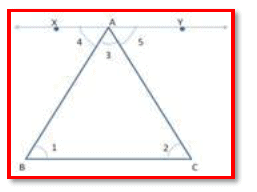Exterior angle property:

An exterior angle of a triangle is equal to the sum of its opposite interior angles.

Eg: In the figure here, ∠4 is called the exterior angle to triangle ABC, and ∠4 = ∠1 + ∠2.

The sum of the lengths of any two sides of a triangle is greater than the third side. In triangle ABC,

Page 23

BC + CA > AB
CA + AB > BC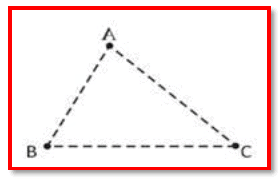In a right-angled triangle, the side opposite the right angle is called the hypotenuse, and the other two sides are called its legs.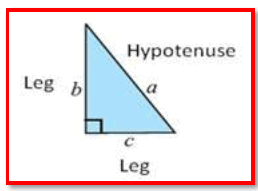Pythagorean theorem:

In a right-angled triangle, the square of the hypotenuse is equal to the sum of the squares of the other two legs. a2 = b2 + c2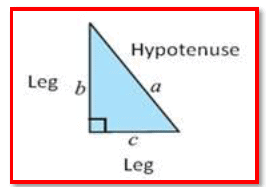Converse:

If the Pythagoras property holds, then the triangle must be right-angled. That is, if there is a triangle such that the sum of the squares on two of its sides is equal to the square of the third side, then it must be a right-angled triangle.

Offer running on EduRev: Apply code STAYHOME200 to get INR 200 off on our premium plan EduRev Infinity!

,

,

,

,

,

,

,

,

,

,

,

,

,

,

,

,

,

,

,

,

,

,

,

,

,

,

,

;